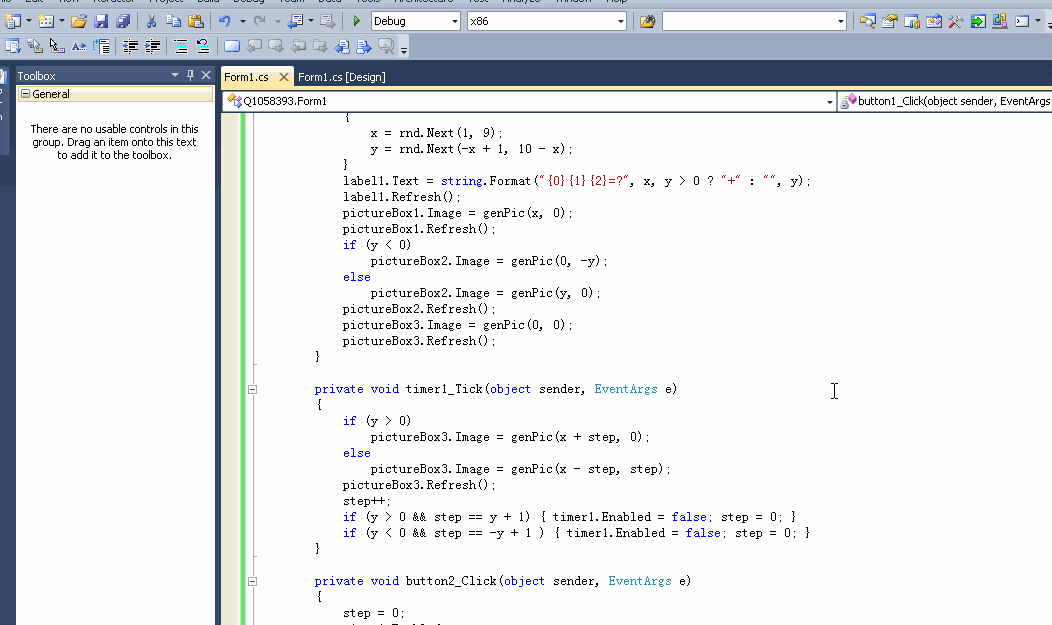2个回答

# 给你做了一个，采纳本回答留下email发给你``````using System;
using System.Collections.Generic;
using System.ComponentModel;
using System.Data;
using System.Drawing;
using System.Linq;
using System.Text;
using System.Windows.Forms;
using Q1058393.Properties;

namespace Q1058393
{
public partial class Form1 : Form
{

private int x = 0;

private int y = 0;

private int step = 0;

private Image genPic(int a, int b)
{
Bitmap bmp = new Bitmap((a + b) * 60 + 10, 60);
Graphics g = Graphics.FromImage(bmp);
var graybmp = ToGray(Resources.Image1);
for (int i = 0; i < a; i++)
{
g.DrawImage(Resources.Image1, i * 60, 5);
}
for (int i = 0; i < b; i++)
{
g.DrawImage(graybmp, (i + a) * 60, 5);
}
return bmp;
}

private Bitmap ToGray(Bitmap bmp)
{
Bitmap b1 = new Bitmap(bmp.Width, bmp.Height);
for (int i = 0; i < bmp.Width; i++)
{
for (int j = 0; j < bmp.Height; j++)
{
Color color = bmp.GetPixel(i, j);
int gray = (int)(color.R * 0.3 + color.G * 0.59 + color.B * 0.11);
Color newColor = Color.FromArgb(gray, gray, gray);
b1.SetPixel(i, j, newColor);
}
}
return b1;
}

public Form1()
{
InitializeComponent();
}

private void button1_Click(object sender, EventArgs e)
{
Random rnd = new Random();
y = 0;
while (y == 0)
{
x = rnd.Next(1, 9);
y = rnd.Next(-x + 1, 10 - x);
}
label1.Text = string.Format("{0}{1}{2}=?", x, y > 0 ? "+" : "", y);
label1.Refresh();
pictureBox1.Image = genPic(x, 0);
pictureBox1.Refresh();
if (y < 0)
pictureBox2.Image = genPic(0, -y);
else
pictureBox2.Image = genPic(y, 0);
pictureBox2.Refresh();
pictureBox3.Image = genPic(0, 0);
pictureBox3.Refresh();
}

private void timer1_Tick(object sender, EventArgs e)
{
if (y > 0)
pictureBox3.Image = genPic(x + step, 0);
else
pictureBox3.Image = genPic(x - step, step);
pictureBox3.Refresh();
step++;
if (y > 0 && step == y + 1) { timer1.Enabled = false; step = 0; }
if (y < 0 && step == -y + 1 ) { timer1.Enabled = false; step = 0; }
}

private void button2_Click(object sender, EventArgs e)
{
step = 0;
timer1.Enabled = true;
label1.Text = string.Format("{0}{1}{2}={3}", x, y > 0 ? "+" : "", y, x+y);
}

}
}

``````